Determine the points in

(i) xy-plane

(ii) yz-plane and

(iii) zx-plane which are equidistant from the points A(1, -1, 0), B(2, 1, 2) and C(3, 2, -1).

Asked by Sakshi | 1 year ago |  39

##### Solution :-

Given:

The points A(1, -1, 0), B(2, 1, 2) and C(3, 2, -1)

(i) xy-plane

We know z = 0 in xy-plane.

So let P(x, y, 0) be any point in xy-plane

According to the question:

PA = PB = PC

PA2 = PB2 = PC2

By using the formula,

The distance between any two points (a, b, c) and (m, n, o) is given by,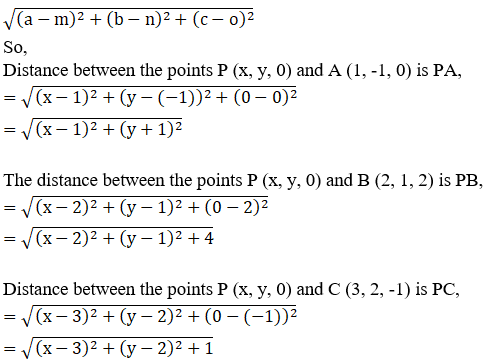We know PA2 = PB2

So, (x – 1)2+ (y + 1)2 = (x – 2)2 + (y – 1)2 + 4

x2+ 1 – 2x + y2 + 1 + 2y = x2+ 4 – 4x + y2 + 1 – 2y + 4

– 2x + 2 + 2y = 9 – 4x – 2y

– 2x + 2 + 2y – 9 + 4x + 2y = 0

2x + 4y – 7 = 0

2x = – 4y + 7……………………(1)

Since, PA2 = PC2

So, (x – 1)2+ (y + 1)2 = (x – 3)2 + (y – 2)2 + 1

x2+ 1 – 2x + y2 + 1 + 2y = x2+ 9 – 6x + y2 + 4 – 4y + 1

– 2x + 2 + 2y = 14 – 6x – 4y

– 2x + 2 + 2y – 14 + 6x + 4y = 0

4x + 6y – 12 = 0

2(2x + 3y – 6) = 0

Now substitute the value of 2x (obtained in equation (1)), we get

7 – 4y + 3y – 6 = 0

– y + 1 = 0

y = 1

By substituting the value of y back in equation (1) we get,

2x = 7 – 4y

2x = 7 – 4(1)

2x = 3

x = $$\dfrac{3}{2}$$

The point P ($$\dfrac{3}{2}$$, 1, 0) in xy-plane is equidistant from A, B and C.

(ii) yz-plane

We know x = 0 in yz-plane.

Let Q(0, y, z) any point in yz-plane

According to the question:

QA = QB = QC

QA2 = QB2 = QC2

By using the formula,

The distance between any two points (a, b, c) and (m, n, o) is given by,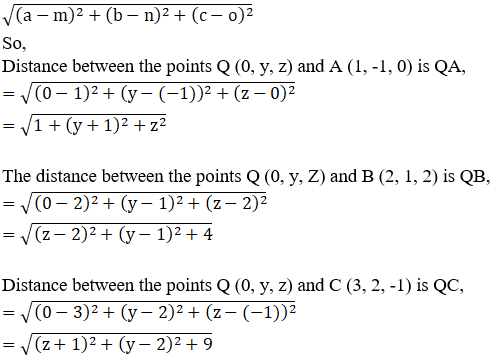We know, QA2 = QB2

So, 1 + z2+ (y + 1)2 = (z – 2)2 + (y – 1)2 + 4

z2+ 1 + y2 + 1 + 2y

= z2+ 4 – 4z + y2 + 1 – 2y + 4

2 + 2y = 9 – 4z – 2y

2 + 2y – 9 + 4z + 2y = 0

4y + 4z – 7 = 0

4z = –4y + 7

z = $$\dfrac{ [–4y + 7]}{4}$$ …. (1)

Since, QA2 = QC2

So, 1 + z2+ (y + 1)2 = (z + 1)2 + (y – 2)2 + 9

2+ 1 + y2 + 1 + 2y = z2+ 1 + 2z + y2 + 4 – 4y + 9

2 + 2y = 14 + 2z – 4y

2 + 2y – 14 – 2z + 4y = 0

–2z + 6y – 12 = 0

2(–z + 3y – 6) = 0

Now, substitute the value of z [obtained from (1)] we get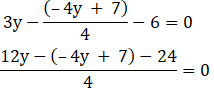12y + 4y – 7 – 24 = 0

16y – 31 = 0

y = $$\dfrac{31}{16}$$

Substitute the value of y back in equation (1), we get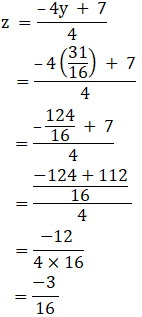$$\dfrac{-3}{16}$$

The point Q (0, $$\dfrac{31}{16}$$, $$\dfrac{-3}{16}$$) in yz-plane is equidistant from A, B and C.

(iii) zx-plane

We know y = 0 in xz-plane.

Let R(x, 0, z) any point in xz-plane

According to the question:

RA = RB = RC

RA2 = RB2 = RC2

By using the formula,

The distance between any two points (a, b, c) and (m, n, o) is given by,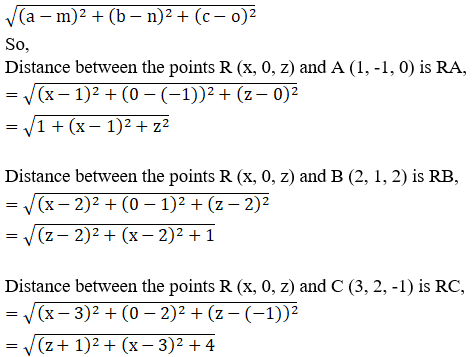We know, RA2 = RB2

So, 1 + z2+ (x – 1)2 = (z – 2)2 + (x – 2)2 + 1

z2+ 1 + x2 + 1 – 2x = z2+ 4 – 4z + x2 + 4 – 4x + 1

2 – 2x = 9 – 4z – 4x

2 + 4z – 9 + 4x – 2x = 0

2x + 4z – 7 = 0

2x = –4z + 7……………………………(1)

Since, RA2 = RC2

So, 1 + z2+ (x – 1)2 = (z + 1)2 + (x – 3)2 + 4

z2+ 1 + x2 + 1 – 2x

= z2+ 1 + 2z + x2 + 9 – 6x + 4

2 – 2x = 14 + 2z – 6x

2 – 2x – 14 – 2z + 6x = 0

–2z + 4x – 12 = 0

2(2x) = 12 + 2z

Substitute the value of 2x [obtained from equation (1)] we get,

2(–4z + 7) = 12 + 2z

–8z + 14 = 12 + 2z

14 – 12 = 8z + 2z

10z = 2

z = $$\dfrac{2}{10}$$

$$\dfrac{1}{5}$$

Now, substitute the value of z back in equation (1), we get

2x = -4z + 7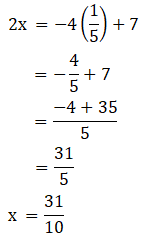The point R ($$\dfrac{31}{10}$$, 0, $$\dfrac{1}{5}$$) in xz-plane is equidistant from A, B and C.

Answered by Aaryan | 1 year ago

### Related Questions

#### A(1, 2, 3), B(0, 4, 1), C(-1, -1, -3) are the vertices of a triangle ABC. Find the point in which the bisector of the

A(1, 2, 3), B(0, 4, 1), C(-1, -1, -3) are the vertices of a triangle ABC. Find the point in which the bisector of the angle ∠BAC meets BC.

#### The mid-points of the sides of a triangle ABC are given by (-2, 3, 5), (4, -1, 7) and (6, 5, 3). Find the coordinates

The mid-points of the sides of a triangle ABC are given by (-2, 3, 5), (4, -1, 7) and (6, 5, 3). Find the coordinates of A, B and C.

#### If the points A(3, 2, -4), B(9, 8, -10) and C(5, 4, -6) are collinear, find the ratio in which C divided AB.

If the points A(3, 2, -4), B(9, 8, -10) and C(5, 4, -6) are collinear, find the ratio in which C divided AB.